# How to Calculate and Solve for the number of Particles in a Substance and Number of Moles | The Calculator Encyclopedia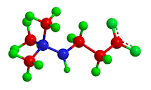The image above represent number of particles in a substance.

To compute for the number of particles in a substance, two essential paramters are needed and these parameters are Avogadro’s constant (h) and number of moles (n).

The formula for calculating the number of particles in a substance:

Number of particles in a substance = n x Avogadro’s constant

Where;
N  = Number of particles in a substance
n = Number of moles
h = Avogadro’s constant

Let’s solve an example:
Find the number of particles in a substance with an Avogadro’s constant of 6.022e+23 and number of moles of 12.

This implies that;
n = Number of moles = 12
h = Avogadro’s constant = 6.022e+23

Number of particles in the substance = 12 x 6.022e+23
Number of particles in the substance = 7.2264e+24

Therefore, the number of particles in the substance is 7.2264e+24 particles.

Nickzom Calculator – The Calculator Encyclopedia is capable of calculating the number of particles in a substance.

To get the answer and workings of the number of particles in a substance using the Nickzom Calculator – The Calculator Encyclopedia. First, you need to obtain the app.

You can get this app via any of these means:

To get access to the professional version via web, you need to register and subscribe for NGN 1,500 per annum to have utter access to all functionalities.
You can also try the demo version via https://www.nickzom.org/calculator

Once, you have obtained the calculator encyclopedia app, proceed to the Calculator Map, then click on Basic Chemistry under ChemistryNow, Click on Number of particles in a substance under Basic Chemistry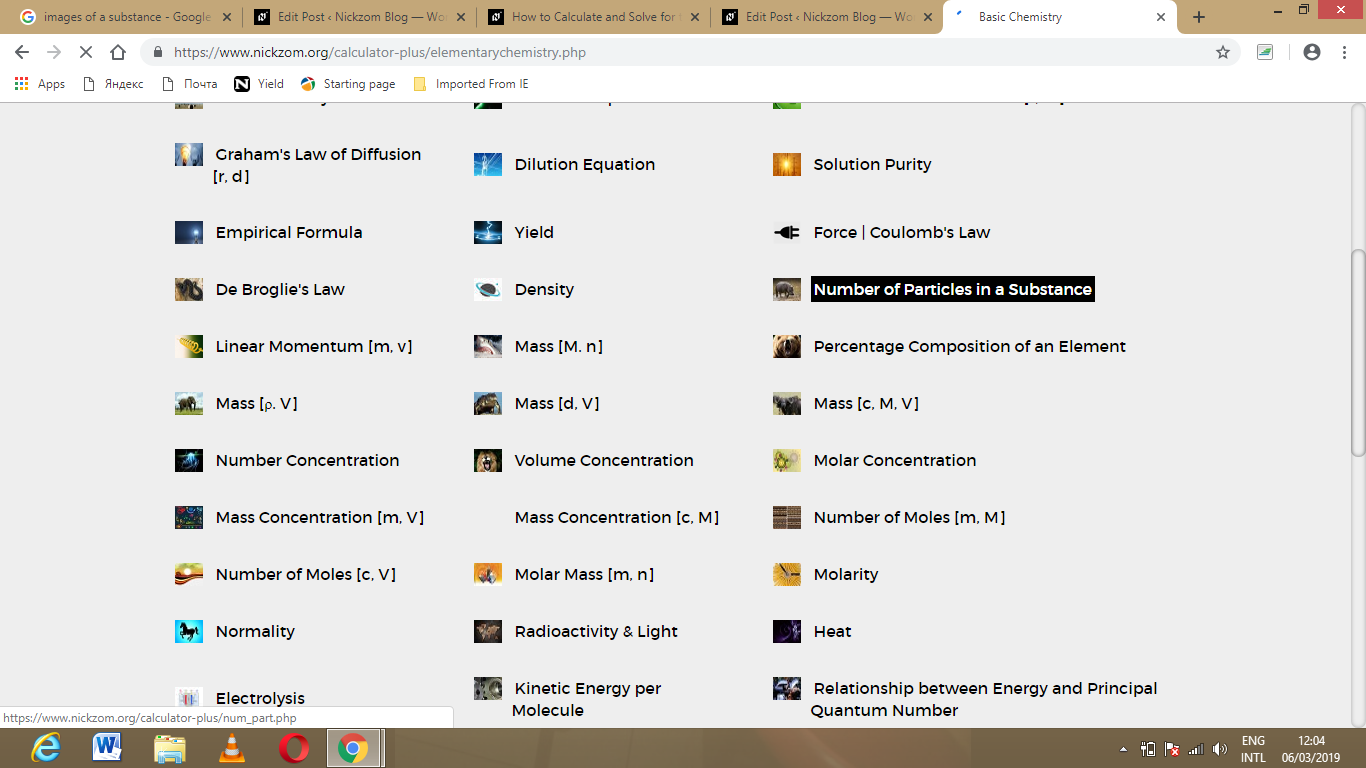The screenshot below displays the page or activity to enter your values, to get the answer for the number of particles in a substance according to the respective parameters which are the Avogadro’s constant (h) and number of moles (n).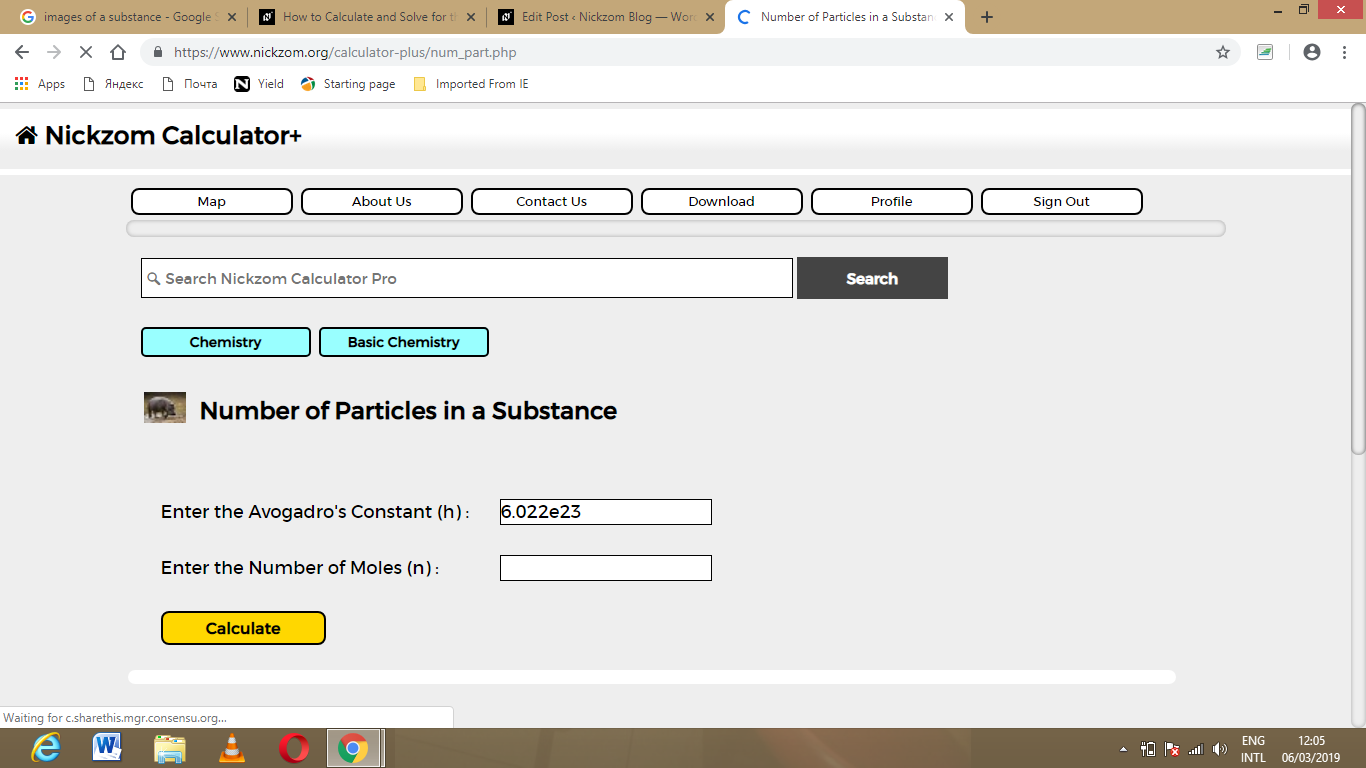Now, enter the values appropriately and accordingly for the parameters as required by the example above where the Avogadro’s constant (h) is 6.022e+23 and number of moles (n) is 12.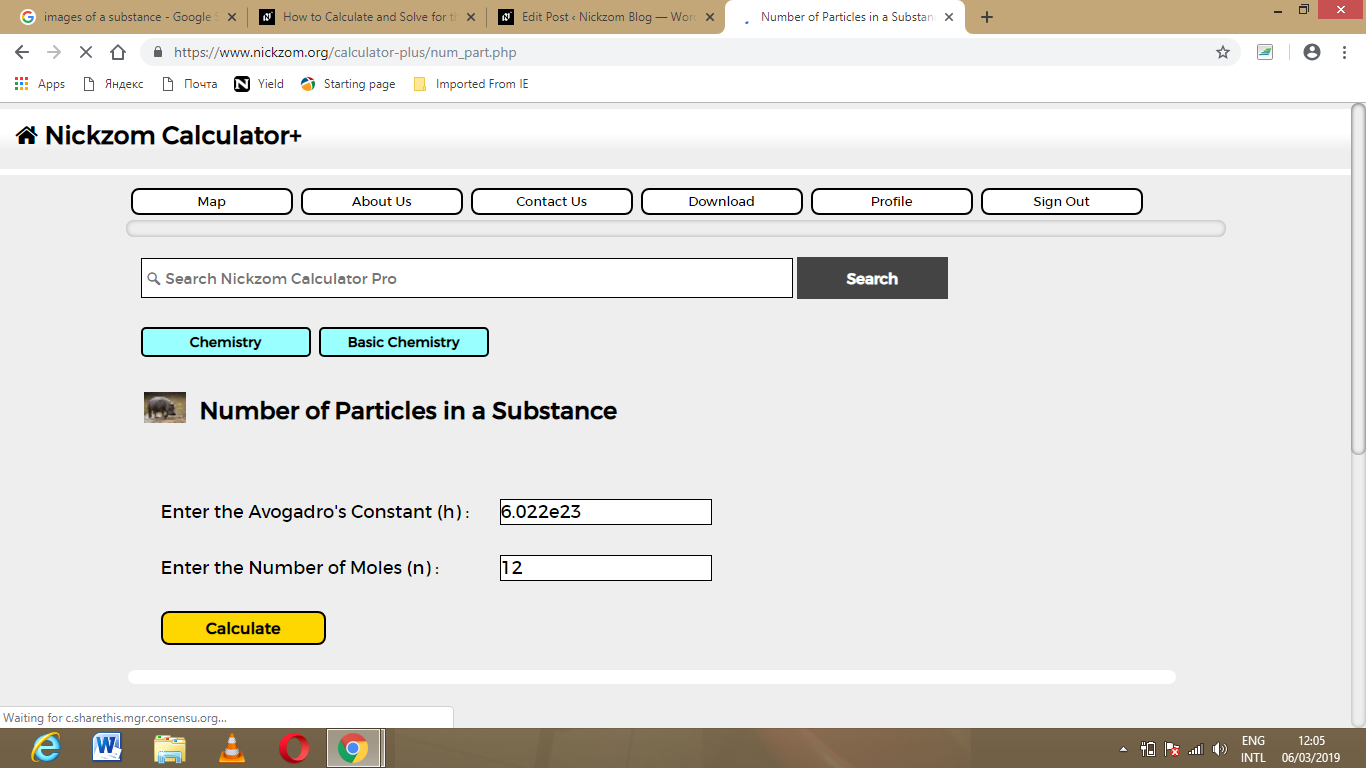Finally, Click on Calculate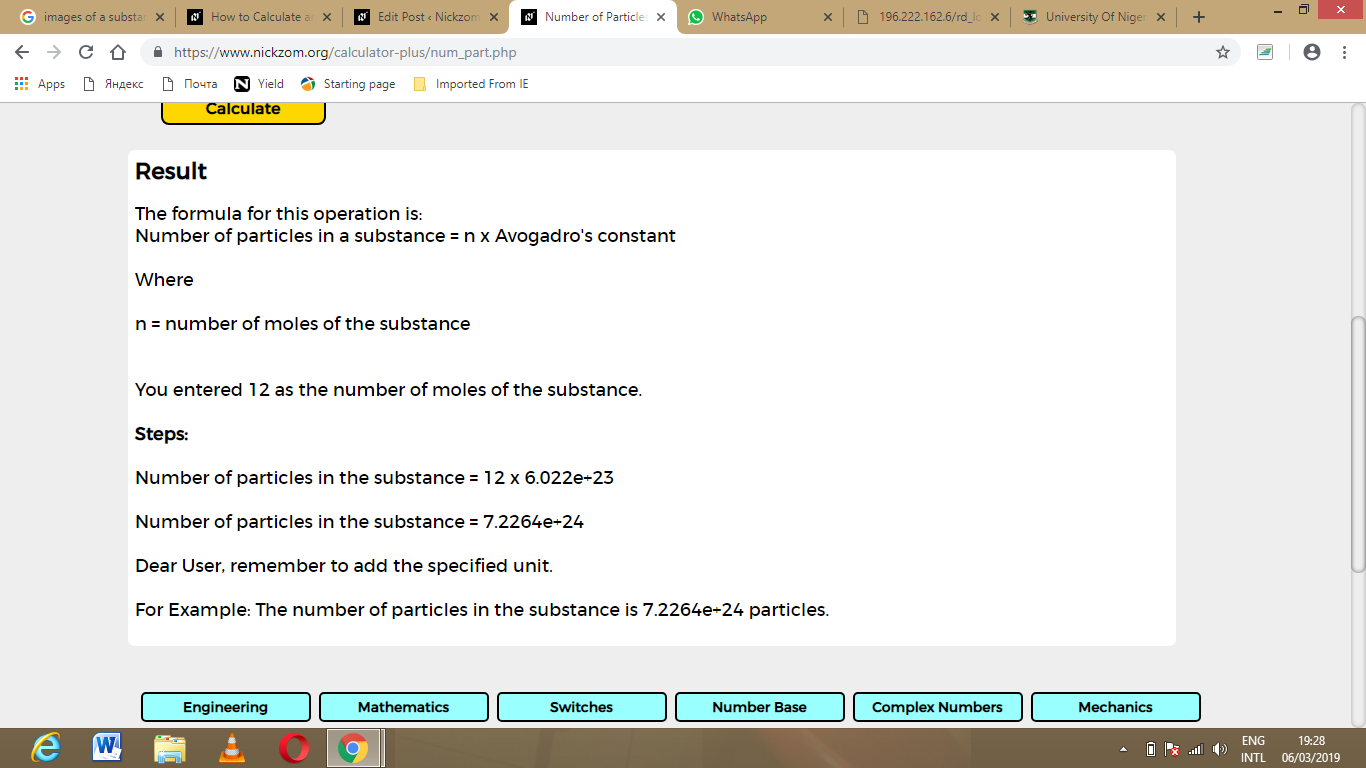As you can see from the screenshot above, Nickzom Calculator – The Calculator Encyclopedia solves for the number of particles in a substance and presents the formula, workings and steps too.